# Go语言的变量有几种类型Go语言是静态类型语言，因此变量（variable）是有明确类型的，编译器也会检查变量类型的正确性。在数学概念中，变量表示没有固定值且可改变的数。但从计算机系统实现角度来看，变量是一段或多段用来存储数据的内存。

• 函数内定义的变量称为局部变量

• 函数外定义的变量称为全局变量

• 函数定义中的变量称为形式参数

【示例】下面的 main() 函数中使用到了局部变量 a、b、c。

```package main
import (
"fmt"
)
func main() {
//声明局部变量 a 和 b 并赋值
var a int = 3
var b int = 4
//声明局部变量 c 并计算 a 和 b 的和
c := a + b
fmt.Printf("a = %d, b = %d, c = %d\n", a, b, c)
}```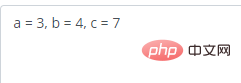```package main
import "fmt"
func main() {
{
name := "HaiCoder"
fmt.Println("Name =", name)
}
}```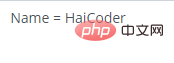```package main
import "fmt"
func main() {
for i := 0; i < 3; i++{
fmt.Print(i)
fmt.Print(" ")
}
fmt.Print(i)
}```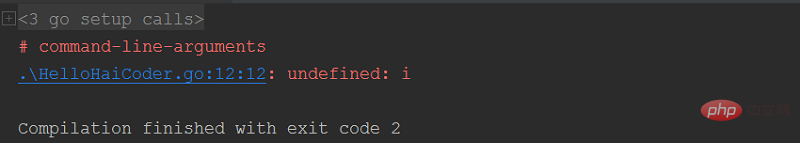【示例】下面代码中，第 6 行定义了全局变量 c。

```package main
import "fmt"
//声明全局变量
var c int
func main() {
//声明局部变量
var a, b int
//初始化参数
a = 3
b = 4
c = a + b
fmt.Printf("a = %d, b = %d, c = %d\n", a, b, c)
}```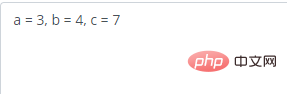```package main
import "fmt"
//声明全局变量
var a float32 = 3.14
func main() {
//声明局部变量
var a int = 3
fmt.Printf("a = %d\n", a)
}```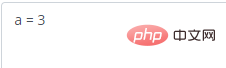【示例】下面代码中第 21 行定义了形式参数 a 和 b。

```package main
import (
"fmt"
)
//全局变量 a
var a int = 13
func main() {
//局部变量 a 和 b
var a int = 3
var b int = 4
fmt.Printf("main() 函数中 a = %d\n", a)
fmt.Printf("main() 函数中 b = %d\n", b)
c := sum(a, b)
fmt.Printf("main() 函数中 c = %d\n", c)
}
func sum(a, b int) int {
fmt.Printf("sum() 函数中 a = %d\n", a)
fmt.Printf("sum() 函数中 b = %d\n", b)
num := a + b
return num
}```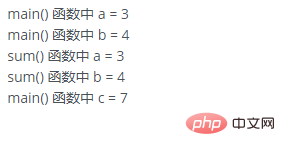【相关推荐：Go视频教程编程教学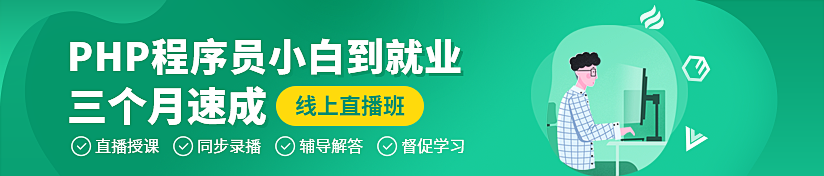#### 相关文章推荐

• go语言的beego是什么• go语言怎么进行强制类型转换• go语言怎么设置时区• Go语言中匿名变量是什么• Go中数值类型有几种
1/1